Скачать презентацию ECON 4120 Applied Welfare Econ Cost Benefit

fab853785239168d1fa14169fd5a3919.ppt

• Количество слайдов: 35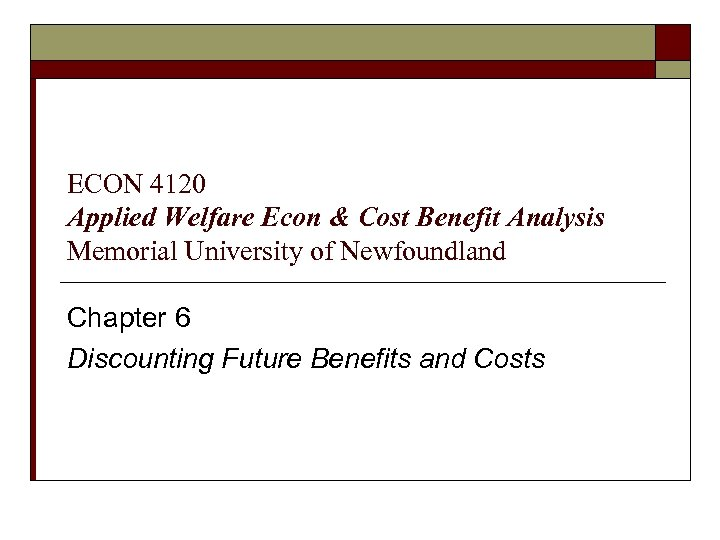ECON 4120 Applied Welfare Econ & Cost Benefit Analysis Memorial University of Newfoundland Chapter 6 Discounting Future Benefits and Costs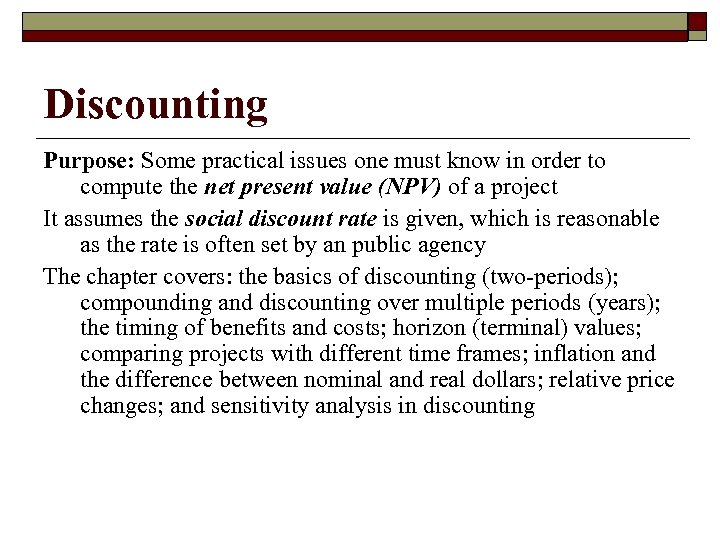Discounting Purpose: Some practical issues one must know in order to compute the net present value (NPV) of a project It assumes the social discount rate is given, which is reasonable as the rate is often set by an public agency The chapter covers: the basics of discounting (two-periods); compounding and discounting over multiple periods (years); the timing of benefits and costs; horizon (terminal) values; comparing projects with different time frames; inflation and the difference between nominal and real dollars; relative price changes; and sensitivity analysis in discounting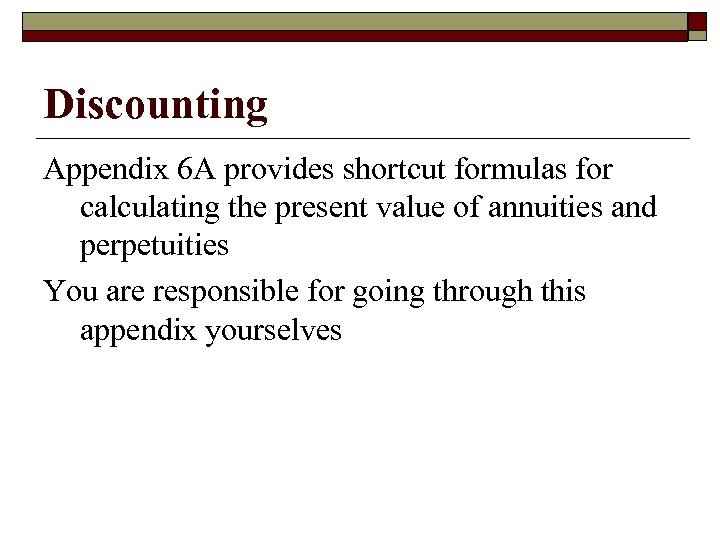Discounting Appendix 6 A provides shortcut formulas for calculating the present value of annuities and perpetuities You are responsible for going through this appendix yourselves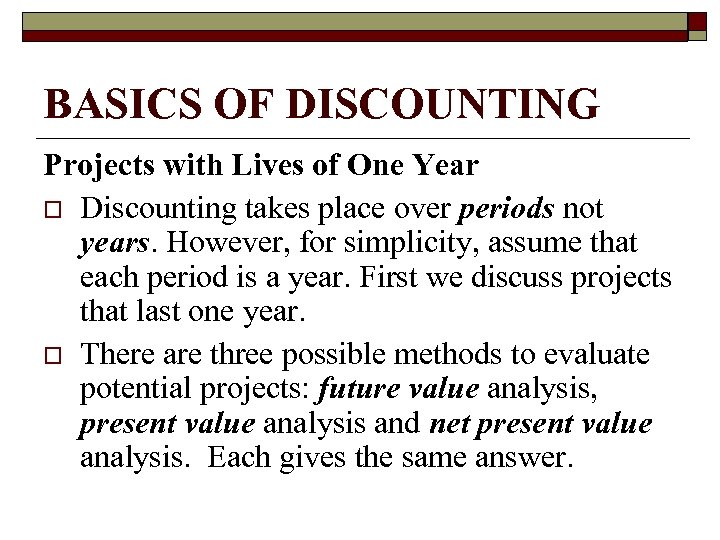BASICS OF DISCOUNTING Projects with Lives of One Year o Discounting takes place over periods not years. However, for simplicity, assume that each period is a year. First we discuss projects that last one year. o There are three possible methods to evaluate potential projects: future value analysis, present value analysis and net present value analysis. Each gives the same answer.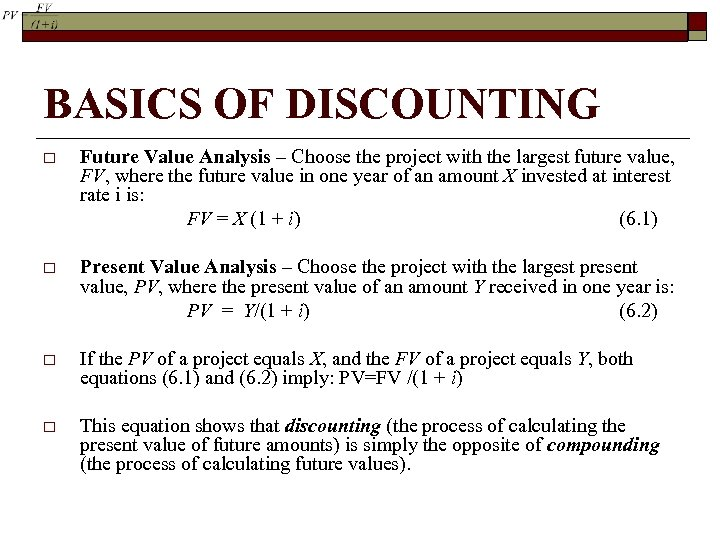BASICS OF DISCOUNTING o Future Value Analysis – Choose the project with the largest future value, FV, where the future value in one year of an amount X invested at interest rate i is: FV = X (1 + i) (6. 1) o Present Value Analysis – Choose the project with the largest present value, PV, where the present value of an amount Y received in one year is: PV = Y/(1 + i) (6. 2) o If the PV of a project equals X, and the FV of a project equals Y, both equations (6. 1) and (6. 2) imply: PV=FV /(1 + i) o This equation shows that discounting (the process of calculating the present value of future amounts) is simply the opposite of compounding (the process of calculating future values).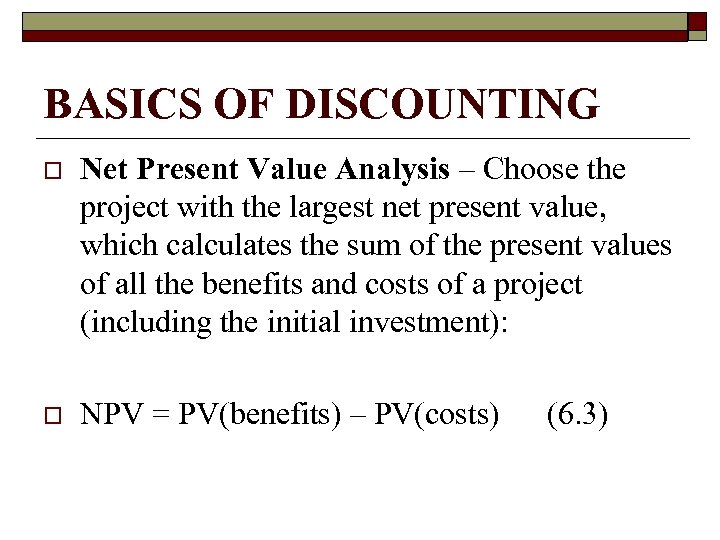BASICS OF DISCOUNTING o Net Present Value Analysis – Choose the project with the largest net present value, which calculates the sum of the present values of all the benefits and costs of a project (including the initial investment): o NPV = PV(benefits) – PV(costs) (6. 3)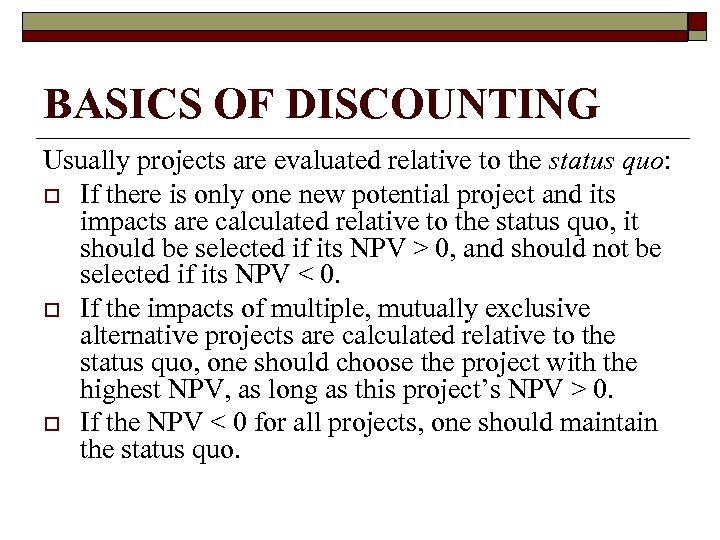BASICS OF DISCOUNTING Usually projects are evaluated relative to the status quo: o If there is only one new potential project and its impacts are calculated relative to the status quo, it should be selected if its NPV > 0, and should not be selected if its NPV < 0. o If the impacts of multiple, mutually exclusive alternative projects are calculated relative to the status quo, one should choose the project with the highest NPV, as long as this project’s NPV > 0. o If the NPV < 0 for all projects, one should maintain the status quo.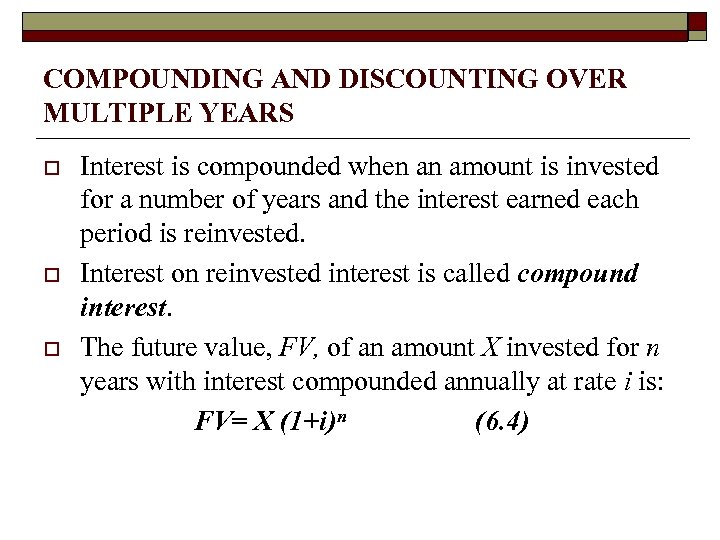COMPOUNDING AND DISCOUNTING OVER MULTIPLE YEARS o o o Interest is compounded when an amount is invested for a number of years and the interest earned each period is reinvested. Interest on reinvested interest is called compound interest. The future value, FV, of an amount X invested for n years with interest compounded annually at rate i is: FV= X (1+i)n (6. 4)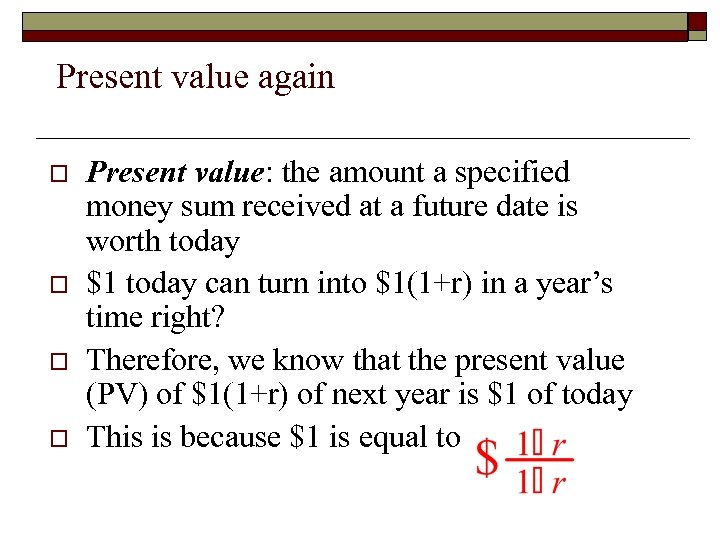Present value again o o Present value: the amount a specified money sum received at a future date is worth today \$1 today can turn into \$1(1+r) in a year’s time right? Therefore, we know that the present value (PV) of \$1(1+r) of next year is \$1 of today This is because \$1 is equal to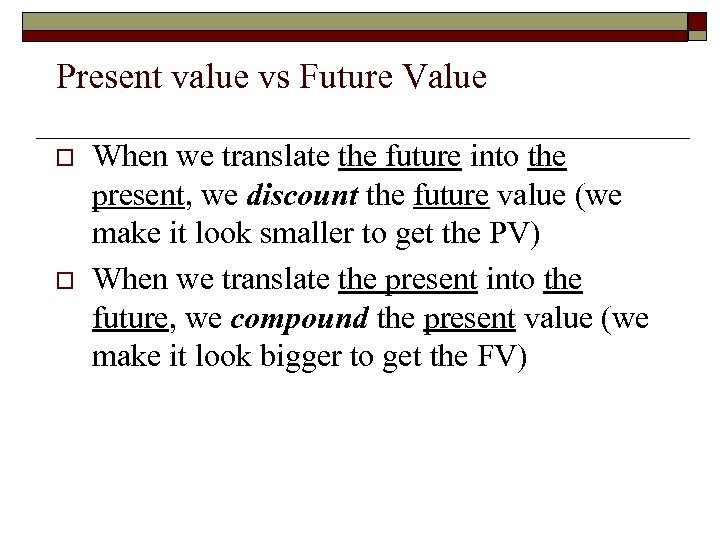Present value vs Future Value o o When we translate the future into the present, we discount the future value (we make it look smaller to get the PV) When we translate the present into the future, we compound the present value (we make it look bigger to get the FV)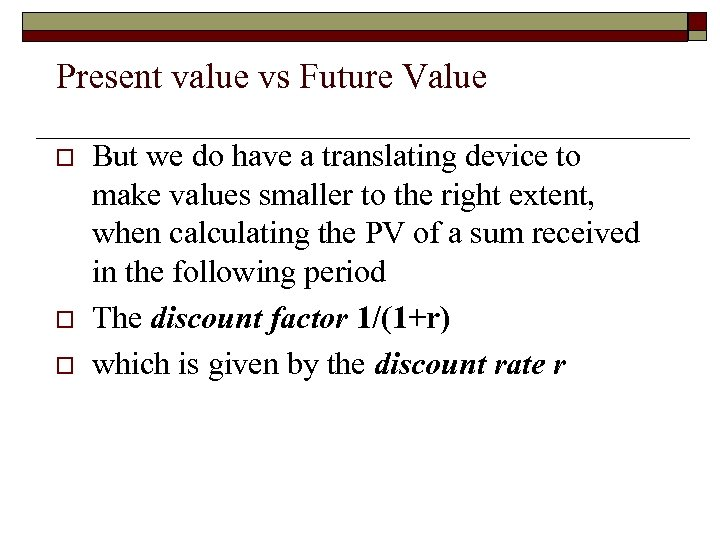Present value vs Future Value o o o But we do have a translating device to make values smaller to the right extent, when calculating the PV of a sum received in the following period The discount factor 1/(1+r) which is given by the discount rate r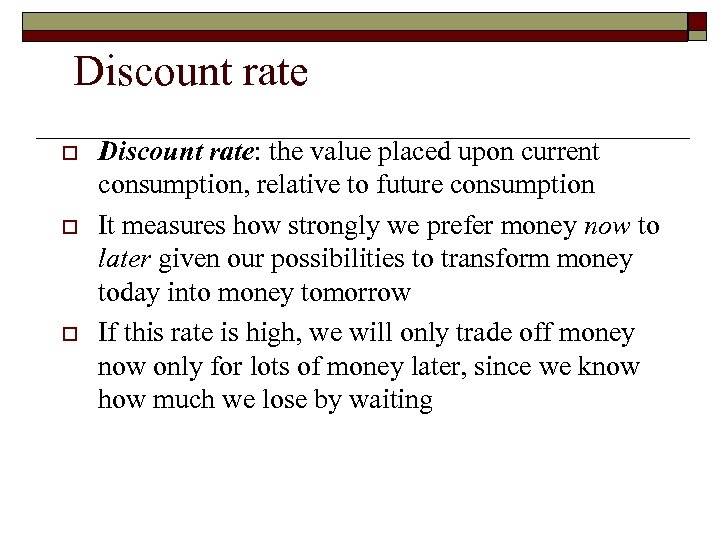Discount rate o o o Discount rate: the value placed upon current consumption, relative to future consumption It measures how strongly we prefer money now to later given our possibilities to transform money today into money tomorrow If this rate is high, we will only trade off money now only for lots of money later, since we know how much we lose by waiting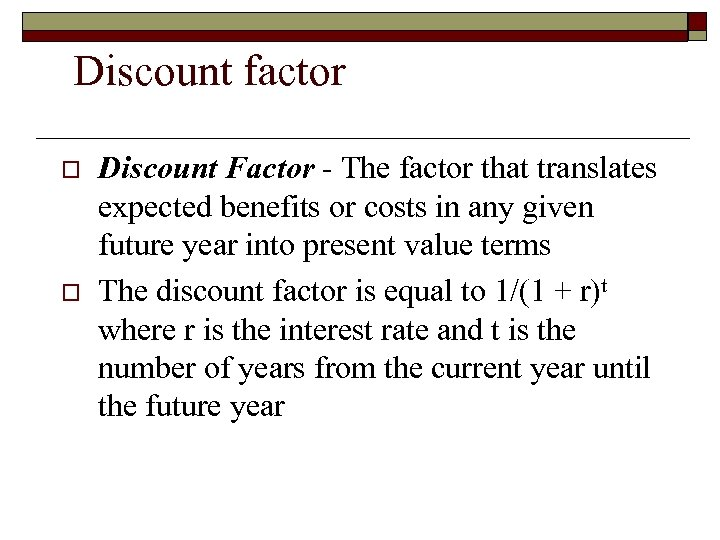Discount factor o o Discount Factor - The factor that translates expected benefits or costs in any given future year into present value terms The discount factor is equal to 1/(1 + r)t where r is the interest rate and t is the number of years from the current year until the future year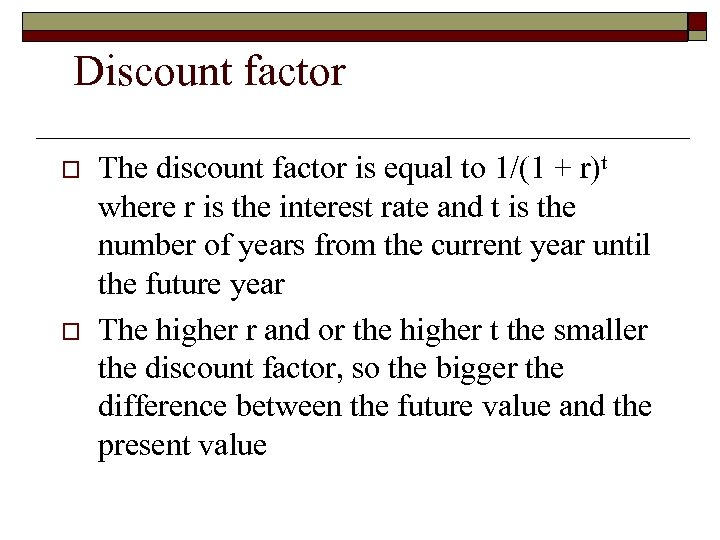Discount factor o o The discount factor is equal to 1/(1 + r)t where r is the interest rate and t is the number of years from the current year until the future year The higher r and or the higher t the smaller the discount factor, so the bigger the difference between the future value and the present value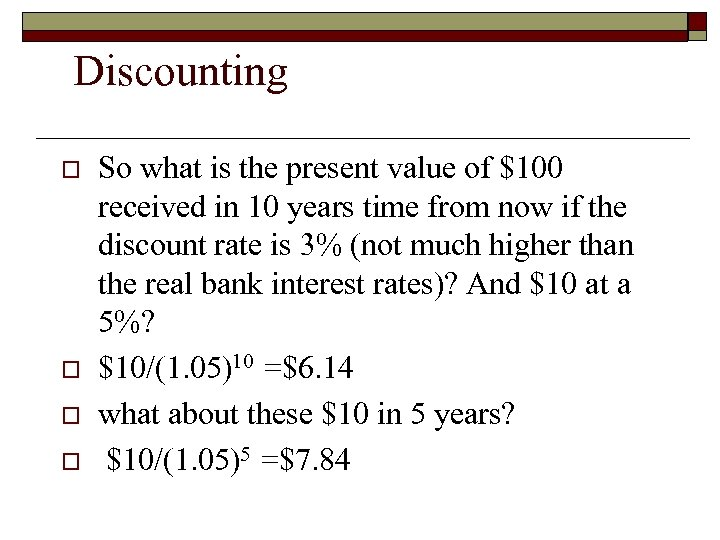Discounting o o So what is the present value of \$100 received in 10 years time from now if the discount rate is 3% (not much higher than the real bank interest rates)? And \$10 at a 5%? \$10/(1. 05)10 =\$6. 14 what about these \$10 in 5 years? \$10/(1. 05)5 =\$7. 84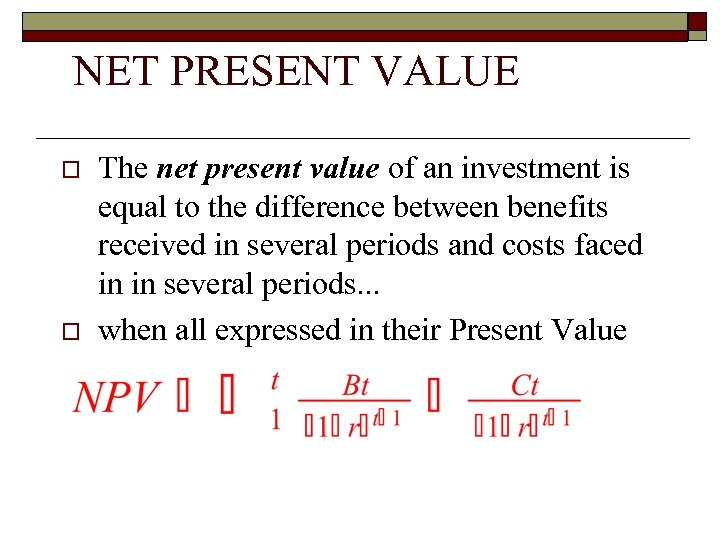NET PRESENT VALUE o o The net present value of an investment is equal to the difference between benefits received in several periods and costs faced in in several periods. . . when all expressed in their Present Value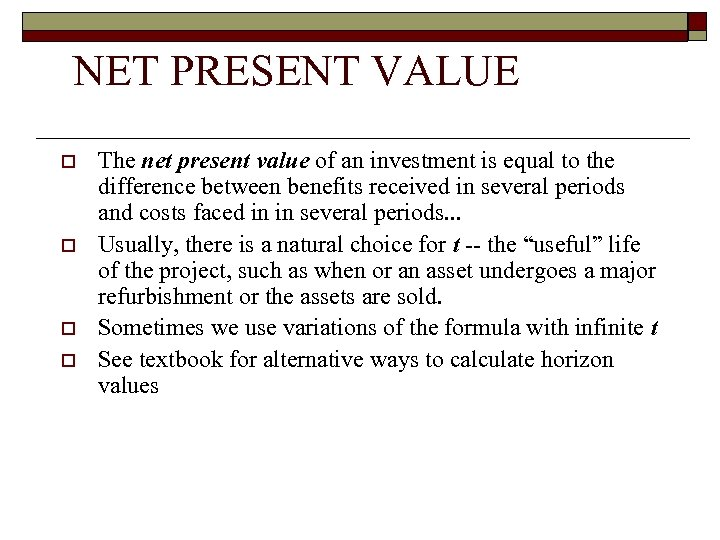NET PRESENT VALUE o o The net present value of an investment is equal to the difference between benefits received in several periods and costs faced in in several periods. . . Usually, there is a natural choice for t -- the “useful” life of the project, such as when or an asset undergoes a major refurbishment or the assets are sold. Sometimes we use variations of the formula with infinite t See textbook for alternative ways to calculate horizon values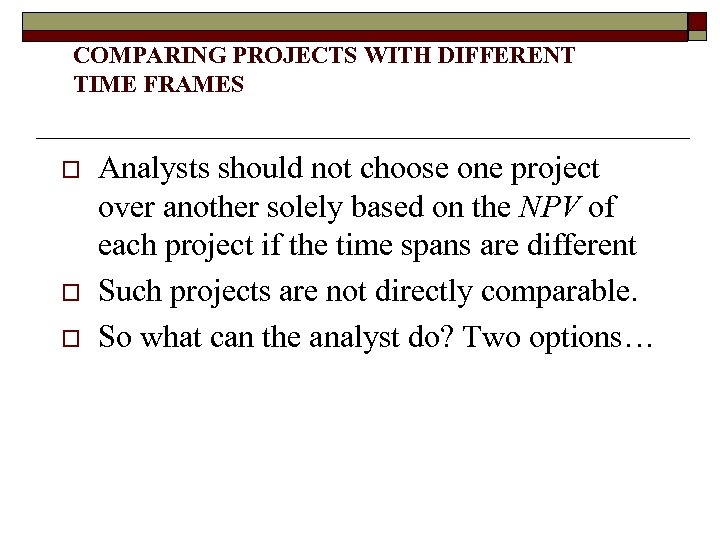COMPARING PROJECTS WITH DIFFERENT TIME FRAMES o o o Analysts should not choose one project over another solely based on the NPV of each project if the time spans are different Such projects are not directly comparable. So what can the analyst do? Two options…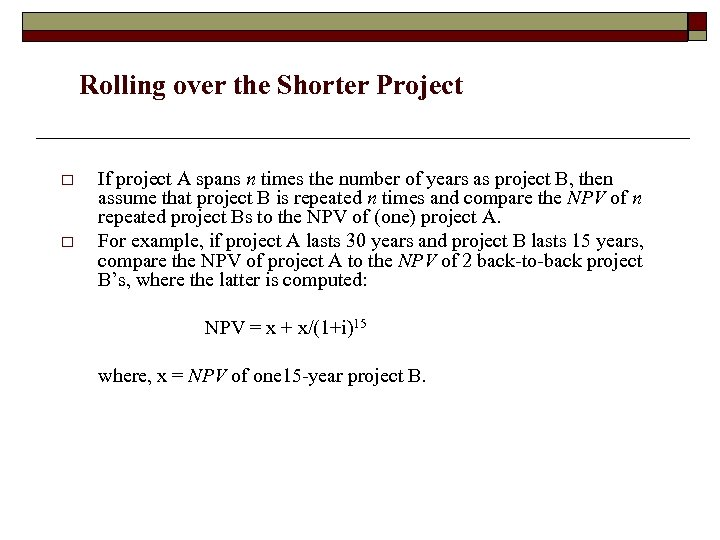Rolling over the Shorter Project o o If project A spans n times the number of years as project B, then assume that project B is repeated n times and compare the NPV of n repeated project Bs to the NPV of (one) project A. For example, if project A lasts 30 years and project B lasts 15 years, compare the NPV of project A to the NPV of 2 back-to-back project B’s, where the latter is computed: NPV = x + x/(1+i)15 where, x = NPV of one 15 -year project B.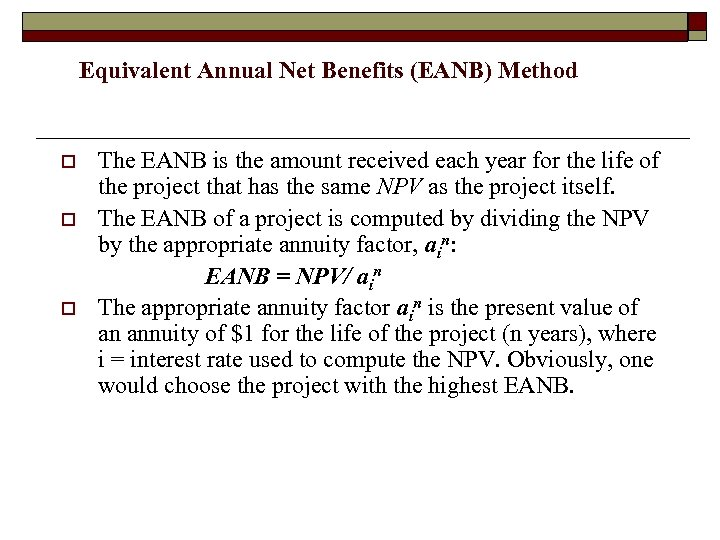Equivalent Annual Net Benefits (EANB) Method o o o The EANB is the amount received each year for the life of the project that has the same NPV as the project itself. The EANB of a project is computed by dividing the NPV by the appropriate annuity factor, ain: EANB = NPV/ ain The appropriate annuity factor ain is the present value of an annuity of \$1 for the life of the project (n years), where i = interest rate used to compute the NPV. Obviously, one would choose the project with the highest EANB.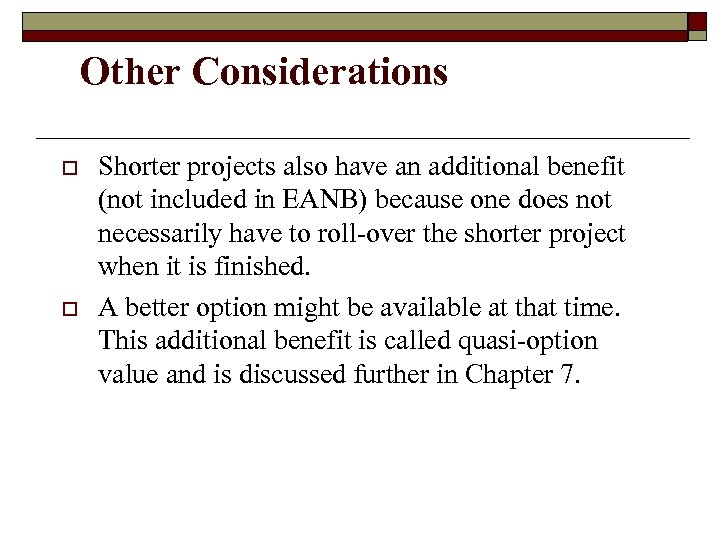Other Considerations o o Shorter projects also have an additional benefit (not included in EANB) because one does not necessarily have to roll-over the shorter project when it is finished. A better option might be available at that time. This additional benefit is called quasi-option value and is discussed further in Chapter 7.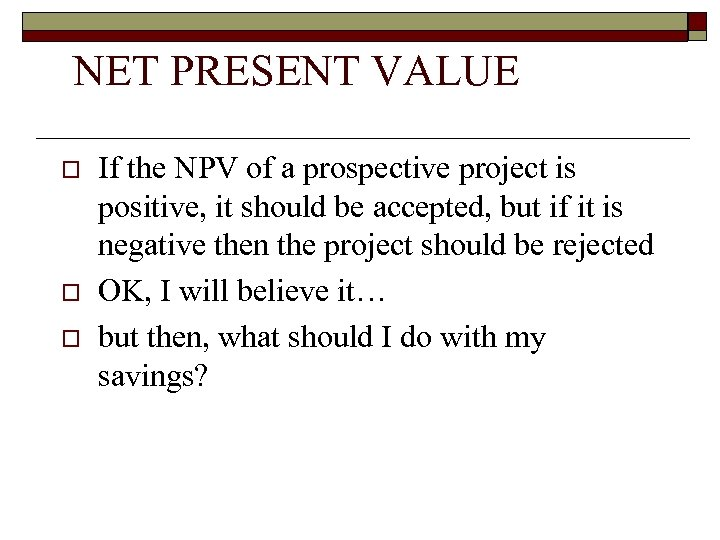NET PRESENT VALUE o o o If the NPV of a prospective project is positive, it should be accepted, but if it is negative then the project should be rejected OK, I will believe it… but then, what should I do with my savings?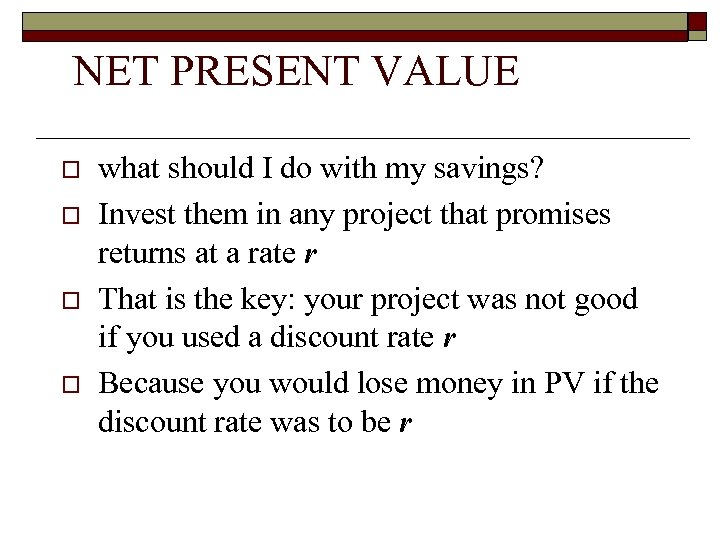NET PRESENT VALUE o o what should I do with my savings? Invest them in any project that promises returns at a rate r That is the key: your project was not good if you used a discount rate r Because you would lose money in PV if the discount rate was to be r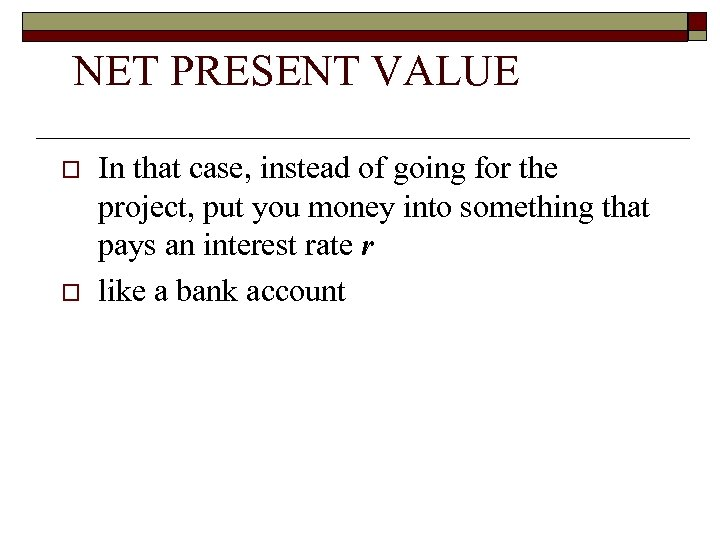NET PRESENT VALUE o o In that case, instead of going for the project, put you money into something that pays an interest rate r like a bank account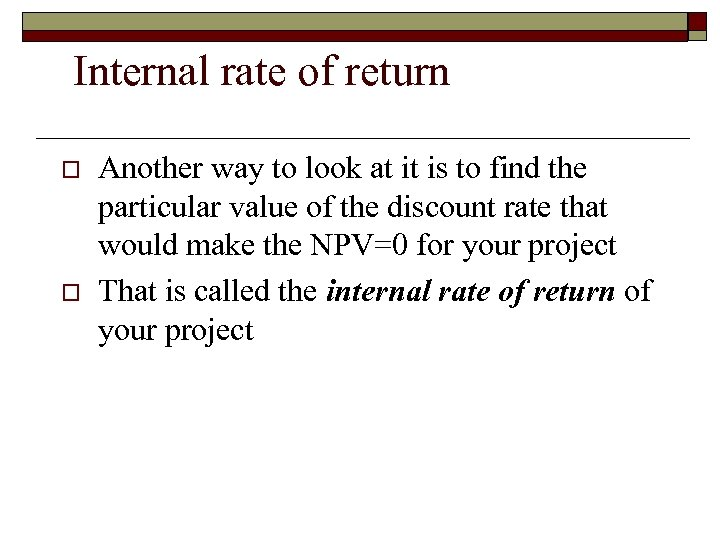Internal rate of return o o Another way to look at it is to find the particular value of the discount rate that would make the NPV=0 for your project That is called the internal rate of return of your project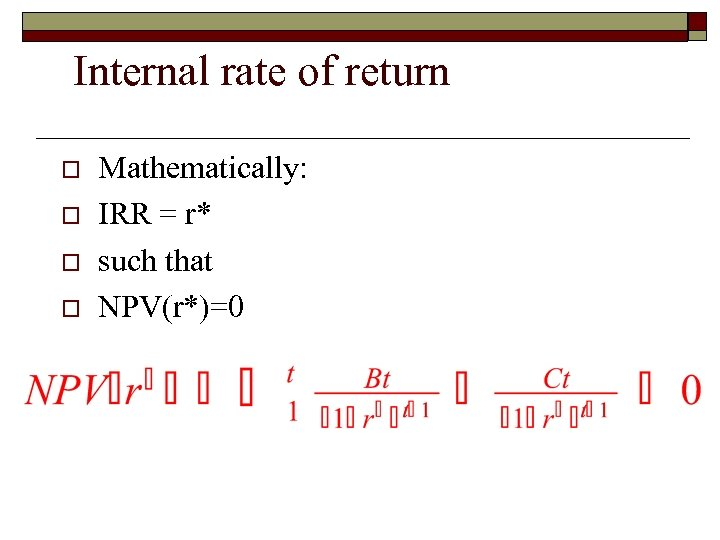Internal rate of return o o Mathematically: IRR = r* such that NPV(r*)=0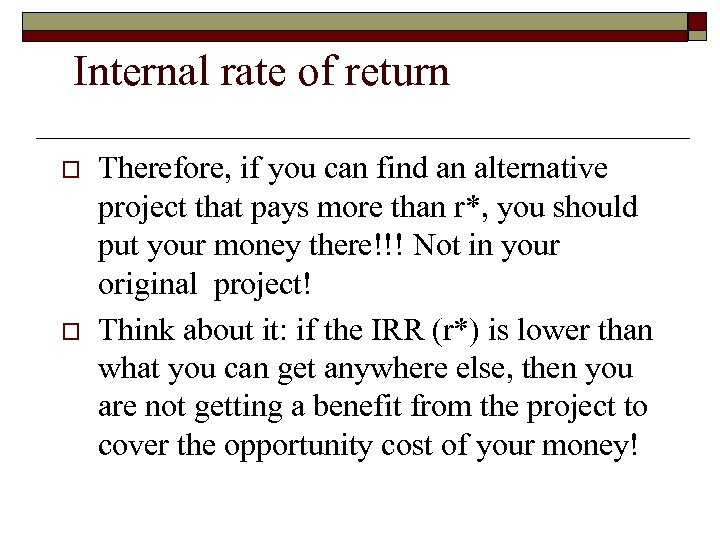Internal rate of return o o Therefore, if you can find an alternative project that pays more than r*, you should put your money there!!! Not in your original project! Think about it: if the IRR (r*) is lower than what you can get anywhere else, then you are not getting a benefit from the project to cover the opportunity cost of your money!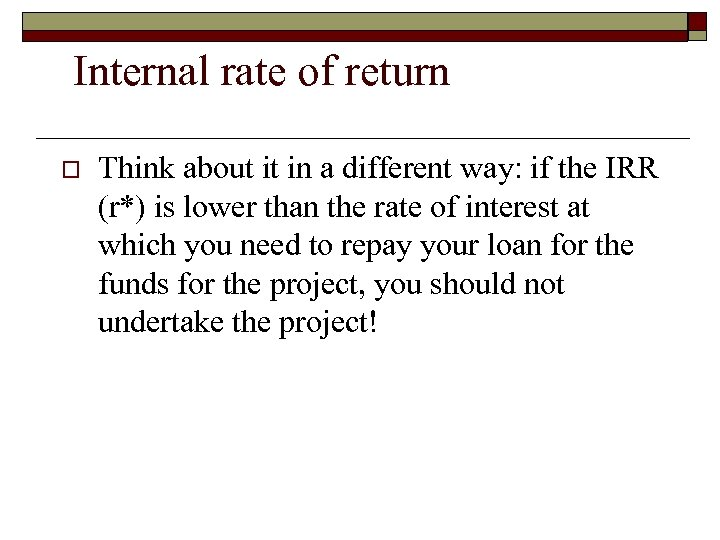Internal rate of return o Think about it in a different way: if the IRR (r*) is lower than the rate of interest at which you need to repay your loan for the funds for the project, you should not undertake the project!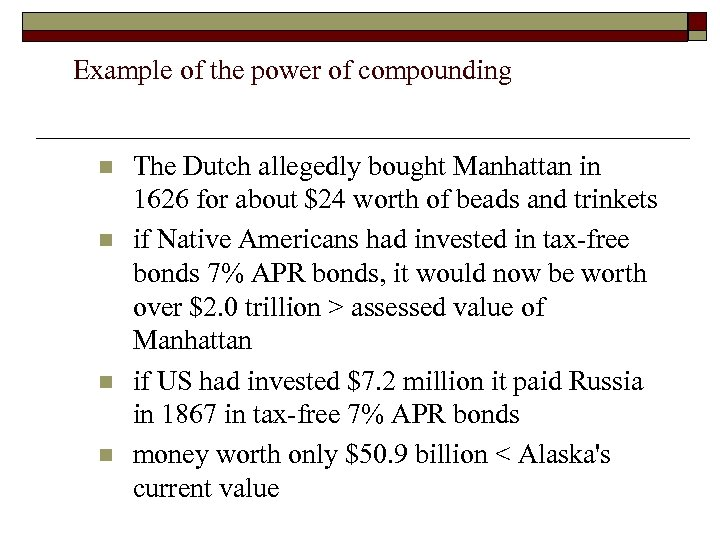Example of the power of compounding n n The Dutch allegedly bought Manhattan in 1626 for about \$24 worth of beads and trinkets if Native Americans had invested in tax-free bonds 7% APR bonds, it would now be worth over \$2. 0 trillion > assessed value of Manhattan if US had invested \$7. 2 million it paid Russia in 1867 in tax-free 7% APR bonds money worth only \$50. 9 billion < Alaska's current value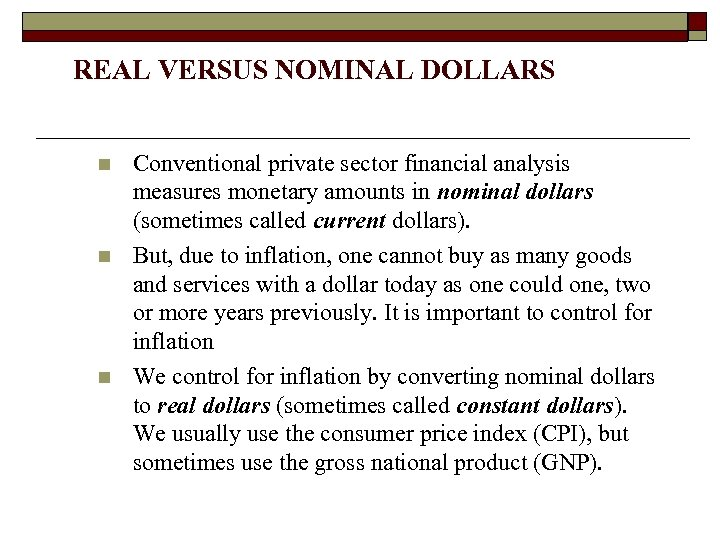REAL VERSUS NOMINAL DOLLARS n n n Conventional private sector financial analysis measures monetary amounts in nominal dollars (sometimes called current dollars). But, due to inflation, one cannot buy as many goods and services with a dollar today as one could one, two or more years previously. It is important to control for inflation We control for inflation by converting nominal dollars to real dollars (sometimes called constant dollars). We usually use the consumer price index (CPI), but sometimes use the gross national product (GNP).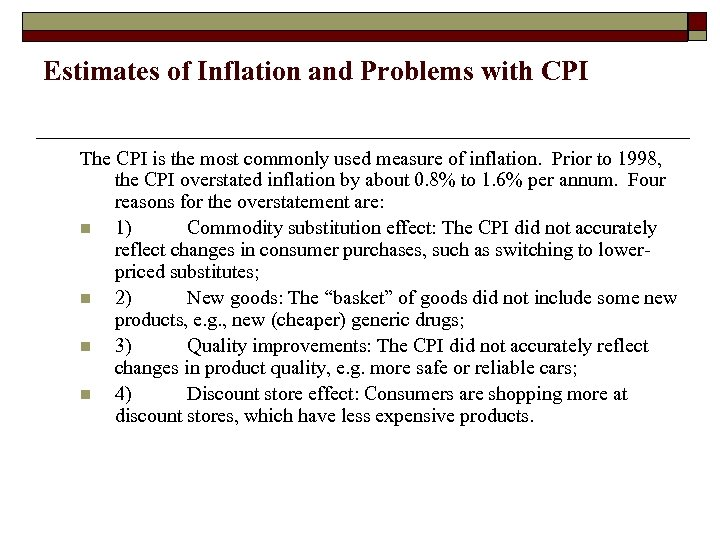Estimates of Inflation and Problems with CPI The CPI is the most commonly used measure of inflation. Prior to 1998, the CPI overstated inflation by about 0. 8% to 1. 6% per annum. Four reasons for the overstatement are: n 1) Commodity substitution effect: The CPI did not accurately reflect changes in consumer purchases, such as switching to lowerpriced substitutes; n 2) New goods: The “basket” of goods did not include some new products, e. g. , new (cheaper) generic drugs; n 3) Quality improvements: The CPI did not accurately reflect changes in product quality, e. g. more safe or reliable cars; n 4) Discount store effect: Consumers are shopping more at discount stores, which have less expensive products.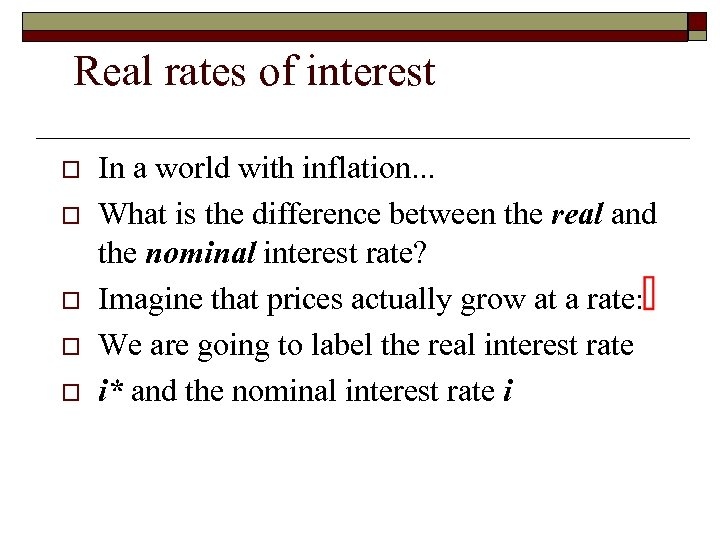Real rates of interest o o o In a world with inflation. . . What is the difference between the real and the nominal interest rate? Imagine that prices actually grow at a rate: We are going to label the real interest rate i* and the nominal interest rate i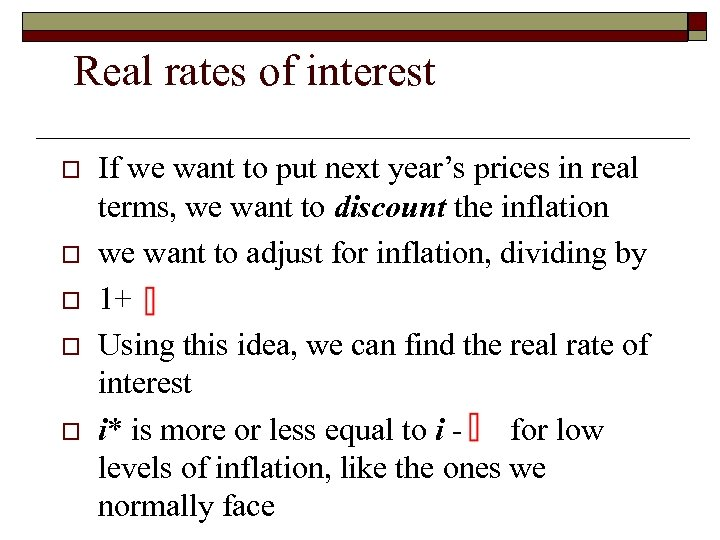Real rates of interest o o o If we want to put next year’s prices in real terms, we want to discount the inflation we want to adjust for inflation, dividing by 1+ Using this idea, we can find the real rate of interest i* is more or less equal to i for low levels of inflation, like the ones we normally face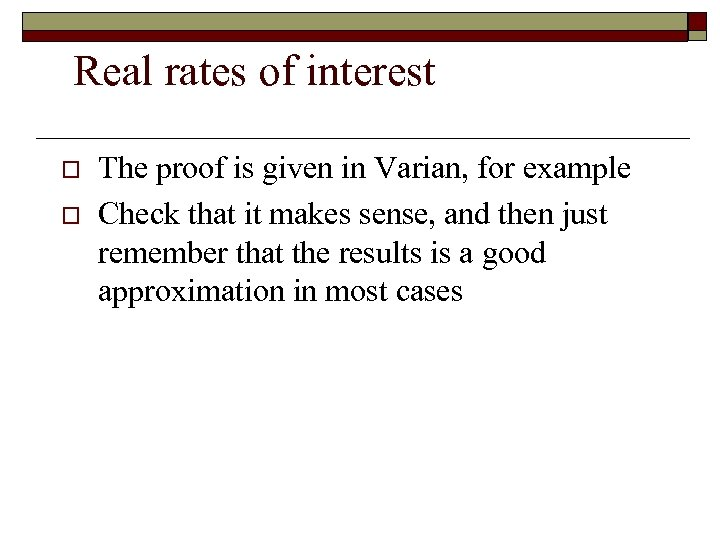Real rates of interest o o The proof is given in Varian, for example Check that it makes sense, and then just remember that the results is a good approximation in most cases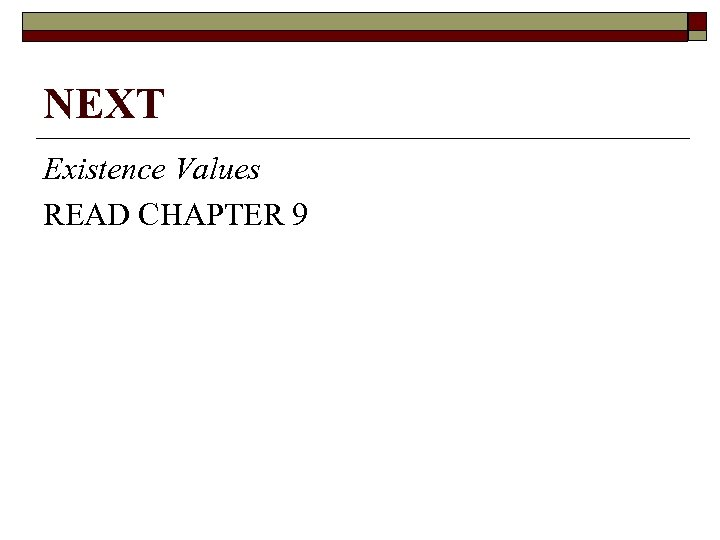NEXT Existence Values READ CHAPTER 9